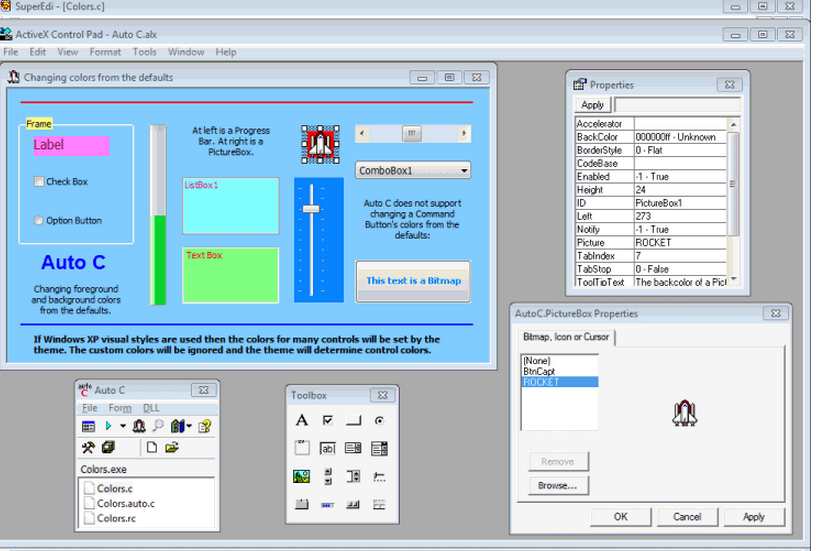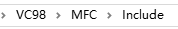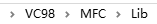• 学计算机的人都知道C语言，而...c语言界面设计代码的操作步骤1、首先打开visual studio c++ 6.0。2、点击菜单栏 文件->新建，弹出新建对话框。3、选择 win32 Applicantion, 工程名字命名为win32gui, 路径设置为自...学计算机的人都知道C语言，而这也是非常常用的一种计算机编程语言。其实c语言运用得当，完全可以编程很多设计代码，帮助你进行界面设计。今天就让小编为大家介绍c语言界面设计。c语言界面设计代码的操作步骤1、首先打开visual studio c++ 6.0。2、点击菜单栏 文件->新建，弹出新建对话框。3、选择 win32 Applicantion, 工程名字命名为win32gui, 路径设置为自己的，点击确定。4、点击确定后，弹出 Win32 Application 对话框，选择一个典型的“Hello World”程序，点击完成。5、工程创建好之后，项目文件设置。6、编译，运行。7、点击代码区域的ResourseView ，然后找到 Dialog8、选中Dialog, 右键选择插入，弹出插入资源对话框，如下面第二张图所示，选择Dialog,点击 新建按钮，一个对话框就这样建好了。c语言界面设计(图片来自网络)9、新建好的对话框初始样子。10、我们可以选择相应的控件，然后将其拖进对话框，进行界面设计，比如我们先选择静态文本控件，将其拖进对话框，选中对话框上的控件，右键属性，修改控件的标题为“姓名”。11、然后从控件中拖一个编辑控件进对话框，并与静态文本控件保持水平。12、讲解怎么将对话框与菜单关联起来，就是鼠标单击菜单选项时候，弹出该对话框。13、点击资源视图里的Menu->IDC_WIN32GUI,在File下增加一个菜单选项，名字叫做opendialog, ID为ID_MENUITEM32772。14、打开win32gui.cpp，在WndProc消息函数增加对话框响应代码，case ID_MENUITEM32772 :　DialogBox(hInst, (LPCTSTR)IDD_DIALOG1 , hWnd, NULL);break。其中ID_MENUITEM32772 是菜单项的id,IDD_DIALOG1 是对话框id,DialogBox是对话框弹出函数。重新编译运行，点击file下的opendialog，弹出刚刚新建的对话框。一个入门的win32 gui就弄好了。注意事项：1、新建的对话框要有消息处理函数，不然打开了就无法关闭了。2、使用vc6.0创建一个Hello World 的win 32 程序，可以在此基础上修改，添加代码，不用从头写。c语言界面设计(图片来自网络)关于c语言C语言是一门面向过程的、抽象化的通用程序设计语言，广泛应用于底层开发。C语言能以简易的方式编译、处理低级存储器。C语言是仅产生少量的机器语言以及不需要任何运行环境支持便能运行的高效率程序设计语言。尽管C语言提供了许多低级处理的功能，但仍然保持着跨平台的特性，以一个标准规格写出的C语言程序可在包括类似嵌入式处理器以及超级计算机等作业平台的许多计算机平台上进行编译。C语言与C++、Java等面向对象编程语言有所不同。C语言的设计目标是提供一种能以简易的方式编译、处理低级存储器、仅产生少量的机器码以及不需要任何运行环境支持便能运行的编程语言。C语言描述问题比汇编语言迅速、工作量小、可读性好、易于调试、修改和移植，而代码质量与汇编语言相当。C语言一般只比汇编语言代码生成的目标程序效率低10%～20%。因此，C语言可以编写系统软件。当前阶段，在编程领域中，C语言的运用非常之多，它兼顾了高级语言和汇编语言的优点，相较于其它编程语言具有较大优势。计算机系统设计以及应用程序编写是C语言应用的两大领域。同时，C语言的普适较强，在许多计算机操作系统中都能够得到适用，且效率显著。以上就是小编为大家介绍的关于c语言界面设计的相关内容。c语言作为计算机的编程语言，完全可以设计相关的程序，对于界面进行设计和优化，你学会了吗？
展开全文• 完全用C语言实现的五子棋。没有用图形界面，因为不大会…… 但在控制台界面里还算是可以的吧 使计算机具有了一定的人工智能 代码在TXT文本里，复制粘贴就好
• C语言版本的俄罗斯方块代码的主界面。很漂亮，但没有主程序
• ## C语言小案例：登录界面

千次阅读 多人点赞 2020-01-20 22:04:27
案例目的：C语言实现登录界面 实现功能： 1.用户登录：用户名、用户密码登录，进入主菜单 密码正确：进入主菜单 密码错误：重新登录或找回密码 找回密码：输入绑定手机号，输入正确，返回账号信息 2.用户注册...
第二个小案例新鲜出炉啦，小人不才，斗胆将其公之于众，请各位大佬手下留情，也希望给出些许建议，万分感谢！
同是天涯码字猿，共勉，共勉！案例目的：C语言实现登录界面
实现功能：
1.用户登录：用户名、用户密码登录，进入主菜单
密码正确：进入主菜单
密码错误：重新登录或找回密码
找回密码：输入绑定手机号，输入正确，返回账号信息
2.用户注册：注册账号、密码，账户密保，返回登录界面
注册账号：输入用户名：用户名重复，重新输入
输入密码：两次输入密码，密码不同，重新输入
输入验证码：产生验证码，输入验证码，不同重新输入
账户密保：绑定手机号，手机号重复，重新输入手机号

函数声明：

#include<stdio.h>
#include<stdlib.h>
#include<string.h>
#include<time.h>
#include<Ctype.h>
#include<windows.h>
void Exit(void);  //退出函数
void Empty(int a);  //清空返回
void IdentifyingCode(void);  //生成验证码
void Create(void);  //用户注册
int TestaCcount(char ch1);  //注册辅助函数：账号验证
void security(char ch1,char ch2)：  //绑定手机
int TestaPhone(char ch1);  //绑定辅助函数：手机号验证
void Entry();  //登录账号
void FoundPassword (void);   //找回密码

主函数：显示登录界面

int main()
{
int n;
do
{
system("cls");
printf("\n                                                   $登录界面$\n");
printf("\n\n                1.用户注册                         2.用户登录                    3.退出程序\n");
printf("\n^*^请选择:");
scanf("%d",&n);
switch(n)
{
case 1:Create();break;
case 2:Entry();break;
case 3:Exit();break;
default:system("cls");printf("#输入有误#");
}
}while(1);
return 0;
}

注册函数：注册新用户账号信息，显示注册页面

void Create(void)  //用户注册
{
FILE *fp;
char ch1,ch2,ch3;
int k=0,i,h=0,g,n;
if((fp=fopen("account.txt","ab"))==NULL)
{
printf("文件打开失败\n");
exit(-1);
}
system("cls");
do
{
printf("\n                                                       $注册$\n");
printf("\n                                 请输入账号:");
for(i=0;i<10;i++)
{
ch1[i]=getch();
printf("%c",ch1[i]);
}
ch1='\0';
if(TestaCcount(ch1)==0)
{
h=1;
fwrite(ch1,10*sizeof(char),1,fp);
}
else
{
system("cls");
printf("#账号已被注册,请重新输入账号#\n");
}
}while(h==0);
putchar(10);
system("cls");
do
{
do
{
n=0;
g=0;
printf("\n                                                       $注册$\n");
printf("\n                                 请输入密码:");
for(i=0;i<10;i++)
{
ch2[i]=getch();
printf("*");
}
ch2='\0';
for(i=0;ch2[i];i++)
{
if(isalpha(ch2[i]))
g=1;
if(!(('1'<=ch2[i] && ch2[i]<='9') || ('a'<=ch2[i] && ch2[i]<='z') || ('A'<=ch2[i] && ch2[i]<='Z')))
{
system("cls");
printf("\n#密码只能包含数字和字母#");
n=1;
break;
}
}
if(g==0 && n==0)
{
system("cls");
printf("\n#密码应至少包含一个字母#\n");
}
}while(g==0);
putchar(10);
printf("                                 再次输入密码:");
for(i=0;i<10;i++)
{
ch3[i]=getch();
printf("*");
}
ch3='\0';
putchar(10);
if(strcmp(ch2,ch3)==0)
{
k=1;
fwrite(ch2,10*sizeof(char),1,fp);
}
else
{
system("cls");
printf("#两次密码不一致#\n");
}
}while(k==0);
fclose(fp);
security(ch1,ch2);
Empty(1);
return;
}

注册辅助函数：验证账号是否已被注册

int TestaCcount(char ch1)  //账号验证
{
FILE *fp;
int g=0;
char a;
if((fp=fopen("account.txt","rb"))==NULL)
{
printf("文件打开失败\n");
exit(-1);
}
while(feof(fp)==0)
{
a='\0';
if(strcmp(a,ch1)==0) g=1;
}
fclose(fp);
if(g==0)
return 0;
else
return 1;
}

绑定函数：绑定手机号

void security(char ch1,char ch2)  //绑定手机
{
FILE *fp;
char ch3;
int i,k=0;
if((fp=fopen("security.txt","ab"))==NULL)
{
printf("文件打开失败\n");
exit(-1);
}
system("cls");
do
{
printf("\n                                                       $绑定$\n");
printf("\n                                 手机号[10位]:");
for(i=0;i<10;i++)
{
ch3[i]=getch();
printf("%c",ch3[i]);
}
ch3='\0';
if(TestaPhone(ch3)==0)
{
k=1;
fwrite(ch3,10*sizeof(char),1,fp);
fwrite(ch2,10*sizeof(char),1,fp);
fwrite(ch1,10*sizeof(char),1,fp);
}
else
{
system("cls");
printf("#该手机号已被绑定#\n");
}
}while(k==0);
fclose(fp);
putchar(10);
IdentifyingCode();
return;
}

绑定辅助函数：验证手机号是否已被绑定

int TestaPhone(char ch1)  //手机号验证
{
FILE *fp;
int g=0;
char a;
if((fp=fopen("security.txt","rb"))==NULL)
{
printf("文件打开失败\n");
exit(-1);
}
while(feof(fp)==0)
{
a='\0';
if(strcmp(a,ch1)==0) g=1;
}
fclose(fp);
if(g==0)
return 0;
else
return 1;
}

验证码函数：随机生成验证码

void IdentifyingCode(void)     //验证码
{
char ch1,ch2;
int a,i;
srand(time(NULL));
system("cls");
do
{
for(i=0;i<4;i++)
{
a=rand()%9;
switch(a)
{
case 1:ch1[i]='2';break;
case 2:ch1[i]='G';break;
case 3:ch1[i]='a';break;
case 4:ch1[i]='5';break;
case 5:ch1[i]='Q';break;
case 6:ch1[i]='0';break;
case 7:ch1[i]='k';break;
case 8:ch1[i]='7';break;
case 9:ch1[i]='S';break;
case 0:ch1[i]='W';break;
default :ch1[i]='A';
}
}
ch1='\0';
printf("\n                                                       $验证$\n");
printf("\n                                 5秒后生成验证码:");
Sleep(5000);
printf("\n                                                 [%s]\n",ch1);
printf("                                 请输入验证码:");
scanf("%s",ch2);
if(strcmp(ch1,ch2)==0)
break;
else
{
system("cls");
printf("#验证码错误#\n");
}
}while(1);
return;
}

登录函数：登录账号

void Entry()  //登录账号
{
int k=0,i;
char ch1,ch2;
system("cls");
do
{
printf("\n                                                       $登录$\n");
printf("\n                                           账号:");
for(i=0;i<10;i++)
{
ch1[i]=getch();
printf("%c",ch1[i]);
}
ch1='\0';
printf("\n                                           密码:");
for(i=0;i<10;i++)
{
ch2[i]=getch();
printf("*");
}
ch2='\0';
k=1;
else
{
system("cls");
}
}while(k==0);
Empty(2);
return;
}

登录辅助函数：验证输入账号信息是否正确

int TestaPassword(char ch1,char ch2)  //密码验证
{
FILE *fp;
int c=0,g=0,k=0;
char a,b;
if((fp=fopen("account.txt","rb"))==NULL)
{
printf("文件打开失败\n");
exit(-1);
}
while(c==0 && feof(fp)==0)
{
a='\0';
if(strcmp(a,ch1)==0)
{
b='\0';
if(strcmp(b,ch2)==0) c=1;
g=1;
}
}
fclose(fp);
if(g==1 && c==1)
return 1;
else
return 0;
}

登录辅助函数：登录出错选择找回密码或重新登录

void PasswordHelp(void)   //密码错误
{
int n;
system("cls");
do
{
printf("\n                                                   $密码错误$\n");
printf("\n\n                        1.找回密码                                            2.重新登录\n");
printf("\n^*^请选择:");
scanf("%d",&n);
if(n==1)
{
return;
}
if(n==2)
return;
else
{
system("cls");
printf("#输入错误#");
}
}while(n!=1 && n!=2);
return;
}

找回密码函数：通过绑定的手机号找回密码

void FoundPassword (void)    //找回密码
{
FILE *fp;
int g=0,i,n;
char a,b,ch1,c;
if((fp=fopen("security.txt","rb"))==NULL)
{
printf("文件打开失败\n");
exit(-1);
}
system("cls");
do
{
printf("\n                                                   $找回密码$\n");
printf("\n                             输入绑定手机号:");
for(i=0;i<10;i++)
{
ch1[i]=getch();
printf("%c",ch1[i]);
}
ch1='\0';
rewind(fp);
while(feof(fp)==0)
{
a='\0';
if(strcmp(a,ch1)==0)
{
b='\0';
c='\0';
g=1;
}
}
if(g==0)
{
system("cls");
printf("#该手机号未被绑定#");
}
else
{
system("cls");
printf("\n                                                   $找回成功$\n");
printf("\n                           5秒后返回账号信息:\n");
Sleep(5000);
printf("\n                                             账号: %s ",c);
printf("\n                                             密码: %s ",b);
}
}while(g==0);
fclose(fp);
do
{
printf("\n\n  ^*^输入 0 返回界面\n");
scanf("%d",&n);
if(n==0)
return;
else
{
system("cls");
printf("#输入有误#\n\n");
}
}while(n!=0);
system("cls");
return;
}

清屏函数：清空界面，返回主界面

void Empty(int a)   //清空返回
{
int n;
system("cls");
do
{
if(a==1)
{
printf("\n                                                   $注册成功$\n");
printf("\n^*^输入 0 返回界面\n");
}
if(a==2)
{
printf("\n                                                   $登录成功$\n");
printf("\n^*^输入 0 进入主菜单\n");
}
scanf("%d",&n);
if(n==0)
return;
else
{
system("cls");
printf("#输入有误#\n\n");
}
}while(n!=0);
}

退出函数：退出程序

void Exit(void)
{
system("cls");
printf("\n\n\n                                     ^*^感谢使用手机模拟系统，祝您生活愉快!\n\n\n\n\n\n\n\n\n\n\n\n\n\n\n\n\n\n\n\n\n\n\n\n\n\n");
exit(-1);
}
注：1.程序通过建立文件，将账号信息写入所建文件，需要时从文件中读出所需信息，本程序共建立两个文件，分别是：account.txt 文件和 security.txt 文件，其中account.txt 文件储存注册的账号与密码，security.txt 文件储存绑定的手机号、账号以及密码。2.system(“cls”);为清屏函数。3.srand(“time(NULL)”);和rand();为随机数函数；
小小推荐：
C语言案例系列：
C语言小案例：学生管理系统1.0版
前端案例系列：
前端小案例：登录页面
撕烂数据爆锤算法系列：
撕烂数据爆锤算法：单链表
撕烂数据爆锤算法：循环链表
刚接触这方面的东西，有很多不解和疑问，希望大家能够多多支持，也希望我能在编程之路上越走越远，哈哈！


展开全文• 本文借助easyx库函数（提供下载链接），很方便地用C语言实现了鼠标、按钮、进度条、坐标尺等操作，为普通C程序的友好人机交互界面提供了一种简单便捷的方案。
目录0.引言1.素材准备2.编程2.1.创建你的界面2.2.创建按钮2.3.鼠标操作2.3.1.单击特效2.3.2.光标感应2.3.3.进度条3.完整代码及效果
0.引言
$\qquad$看了CSDN上很多关于C程序图形化界面的介绍，有的代码繁琐难解，不方便调试修改；有的不够详细。本文提供的代码简单、易于移植、容易理解，望急需使用C语言制作图形化界面的朋友采纳。
$\qquad$对easyx尚不熟悉的朋友不需要担心，我敢打包票它只需10分钟就可以上手，而它为你节省的时间可能是3个小时甚至更多。关于easyx的简单应用请参考一篇我以前写的关于C程序可视化的博文。
→【C语言实现动画控制】←
$\qquad$本文的讲解是循序渐进的，读者应该重点关注每个步骤的理解，两步之间代码的变化，即可完全理解本文。
1.素材准备

easyx的下载链接如下：（本文使用的版本是2014冬至版）
注：使用easyx需要注意它兼容的编译器（下载的帮助文件会写），不同的easyx兼容的编译器不同，但总是和visual C++6兼容（和字符编码有关），本文以visual C++6编译器为例书写代码。
easyx的最新英文帮助文档链接（下载2014冬至版会自带中文帮助文档）：
https://docs.easyx.cn/en-us/intro
如果你成功下载了easyx2014冬至版，那么解压后把头文件（easyx.h和graphic.h）和lib文件（amd64）分别放在VC文件夹默认的include文件夹和lib文件夹中。右键你的VC程序，选择打开文件所在位置，然后找到MFC文件夹，友情提供两个文件夹的位置截图。建议编译的C文件以cpp后缀保存。

2.编程
2.1.创建你的界面
$\qquad$创建一个480×360的窗口，我们需要使用initgraph()函数，闲言少叙，让我们直接看一段代码：
#include <graphics.h>              // 引用图形库头文件
#include <conio.h>
#include <stdio.h>
#include <windows.h>				//用到了定时函数sleep()
#include <math.h>

int main()
{
int i;
short win_width,win_height;//定义窗口的宽度和高度
win_width = 480;win_height = 360;
initgraph(win_width,win_height);//初始化窗口（黑屏）
for(i=0;i<256;i+=5)
{
setbkcolor(RGB(i,i,i));//设置背景色，原来默认黑色
cleardevice();//清屏（取决于背景色）
Sleep(15);//延时15ms
}
closegraph();//关闭绘图界面
}


$\qquad$这段代码很容易理解，运行这段程序，就会出现逐渐明亮的屏幕。因为不断刷新背景色为$RGB(i,i,i)$。C语言中的颜色使用十六进制表示的，RGB函数可以将0~255范围内的三个整数三原色转换成这个十六进制。
$\qquad$cleardevice()函数用于清屏，是界面内所有元素都被清空，一般只会在初始化出现。
$\qquad$Sleep()是毫秒级延迟，当然界面变亮时间不一定是准确的15ms×255/5=0.765s，因为其他语句还需要执行时间。
$\qquad$closegraph()：关闭绘图界面。注意，如果初始化了绘图界面但没有在主函数结束前关闭它，可能会引发一些莫名其妙的错误！所以这个函数一定要有！
2.2.创建按钮
$\qquad$我们尝试在界面创建几个按钮，按钮需要的操作是绘制矩形和打印文字。虽然看着简单，但是里面还是有点学问，为了方便大家理解，还是先放上代码和注释。
#include <graphics.h>              // 引用图形库头文件
#include <conio.h>
#include <stdio.h>
#include <windows.h>				//用到了定时函数sleep()
#include <math.h>
int r1[]={30,20,130,60};//输入按钮的矩形参数
int r2[]={170,20,220,60};//运行按钮的矩形参数
int r3[]={260,20,310,60};//退出按钮的矩形参数
int main()
{
int i;
short win_width,win_height;//定义窗口的宽度和高度
win_width = 480;win_height = 360;
initgraph(win_width,win_height);//初始化窗口（黑屏）
for(i=0;i<256;i+=5)
{
setbkcolor(RGB(i,i,i));//设置背景色，原来默认黑色
cleardevice();//清屏（取决于背景色）
Sleep(15);//延时15ms
}
RECT R1={r1,r1,r1,r1};//矩形指针R1
RECT R2={r2,r2,r2,r2};//矩形指针R2
RECT R3={r3,r3,r3,r3};//矩形指针R3
LOGFONT f;//字体样式指针
gettextstyle(&f);					//获取字体样式
_tcscpy(f.lfFaceName,_T("宋体"));	//设置字体为宋体
f.lfQuality = ANTIALIASED_QUALITY;    // 设置输出效果为抗锯齿
settextstyle(&f);                     // 设置字体样式
settextcolor(BLACK);				//BLACK在graphic.h头文件里面被定义为黑色的颜色常量
drawtext("输入参数",&R1,DT_CENTER | DT_VCENTER | DT_SINGLELINE);//在矩形区域R1内输入文字，水平居中，垂直居中，单行显示
drawtext("运行",&R2,DT_CENTER | DT_VCENTER | DT_SINGLELINE);//在矩形区域R2内输入文字，水平居中，垂直居中，单行显示
drawtext("退出",&R3,DT_CENTER | DT_VCENTER | DT_SINGLELINE);//在矩形区域R3内输入文字，水平居中，垂直居中，单行显示
setlinecolor(BLACK);
rectangle(r1,r1,r1,r1);
rectangle(r2,r2,r2,r2);
rectangle(r3,r3,r3,r3);
system("pause");//暂停，为了显示
closegraph();
return 0;
}$\qquad$这里需要特别介绍的是矩形指针$pRect$，它使用句柄RECT定义，并且不可以中途再次赋值。之所以要设置矩形指针了为了打印字体的时候以矩形为边界自动填充。它的格式是RECT r={X1,Y1,X2,Y2}，X1和X2是矩形的左边和右边的横坐标，Y1和Y2是矩形的上边和下边的纵坐标，这一点和rectangle()绘制空心矩形函数参数排列一致。后面的DT_CENTER | DT_VCENTER | DT_SINGLELINE就是描述填充格式的常量。使用drawtext书写文字不需要再计算文字的坐标和设置大小，会方便很多。
$\qquad$LOGFONT是字体样式指针，通过gettextstyle()函数来获取当前的字体类型，再通过settextstyle()函数加以设置。这里只修改了字体的名称和显示质量，还可以修改斜体、下划线等属性，更详细的部分请参考帮助文档。
2.3.鼠标操作
2.3.1.单击特效
$\qquad$作为一个图形化界面的C程序，鼠标操作总不能少吧。在讲解程序前先别着急，简单为大家科普一下鼠标事件：
$\qquad$鼠标是输入设备，只要发生以下的事件，就会暂存在鼠标消息列表中，我们的操作系统就会依次响应列表中的鼠标消息事件，常用的鼠标事件如下：

WM_MOUSEMOVE——鼠标移动
WM_MOUSEWHEEL——鼠标滚轮滚动
WM_LBUTTONDOWN——鼠标左键按下
WM_LBUTTONUP——鼠标左键弹起
WM_LBUTTONDBLCLK——鼠标左键双击
WM_RBUTTONDOWN——鼠标右键按下
WM_RBUTTONUP——鼠标右键弹起
WM_RBUTTONDBLCLK——鼠标左键双击
WM_MBUTTONDOWN——鼠标中键按下
WM_MBUTTONUP——鼠标中键弹起
WM_MBUTTONDBLCLK——鼠标中键双击
$\qquad$我们只需要根据不断获取鼠标消息队列的消息并根据消息依次进行响应即可。

$\qquad$相信大家已经迫不及待了，那么请看下面一个简单的程序。
#include <graphics.h>              // 引用图形库头文件
#include <conio.h>
#include <stdio.h>
#include <windows.h>				//用到了定时函数sleep()
#include <math.h>
int r1[]={30,20,130,60};//输入按钮的矩形参数
int r2[]={170,20,220,60};//运行按钮的矩形参数
int r3[]={260,20,310,60};//退出按钮的矩形参数
int main()
{
int i;
short win_width,win_height;//定义窗口的宽度和高度
win_width = 480;win_height = 360;
initgraph(win_width,win_height);//初始化窗口（黑屏）
for(i=0;i<256;i+=5)
{
setbkcolor(RGB(i,i,i));//设置背景色，原来默认黑色
cleardevice();//清屏（取决于背景色）
Sleep(15);//延时15ms
}
RECT R1={r1,r1,r1,r1};//按钮1的矩形区域
RECT R2={r2,r2,r2,r2};//按钮2的矩形区域
RECT R3={r3,r3,r3,r3};//按钮2的矩形区域
LOGFONT f;
gettextstyle(&f);					//获取字体样式
_tcscpy(f.lfFaceName,_T("宋体"));	//设置字体为宋体
f.lfQuality = ANTIALIASED_QUALITY;    // 设置输出效果为抗锯齿
settextstyle(&f);                     // 设置字体样式
settextcolor(BLACK);				//BLACK在graphic.h头文件里面被定义为黑色的颜色常量
drawtext("输入参数",&R1,DT_CENTER | DT_VCENTER | DT_SINGLELINE);//在矩形区域R1内输入文字，水平居中，垂直居中，单行显示
drawtext("运行",&R2,DT_CENTER | DT_VCENTER | DT_SINGLELINE);//在矩形区域R2内输入文字，水平居中，垂直居中，单行显示
drawtext("退出",&R3,DT_CENTER | DT_VCENTER | DT_SINGLELINE);//在矩形区域R3内输入文字，水平居中，垂直居中，单行显示
setlinecolor(BLACK);
rectangle(r1,r1,r1,r1);
rectangle(r2,r2,r2,r2);
rectangle(r3,r3,r3,r3);
MOUSEMSG m;//鼠标指针
setrop2(R2_NOTXORPEN);//二元光栅——NOT(屏幕颜色 XOR 当前颜色)
while(true)
{
m = GetMouseMsg();//获取一条鼠标消息
if(m.uMsg==WM_LBUTTONDOWN)
{
for(i=0;i<=10;i++)
{
setlinecolor(RGB(25*i,25*i,25*i));//设置圆颜色
circle(m.x,m.y,2*i);
Sleep(25);//停顿2ms
circle(m.x,m.y,2*i);//抹去刚刚画的圆
}
FlushMouseMsgBuff();//清空鼠标消息缓存区
}
}
system("pause");//暂停，为了显示
closegraph();
return 0;
}$\qquad$每点击鼠标以下，应该可以看到鼠标点击处有一个逐渐扩大并淡出的圆（截图无法清晰，在画面的中右侧），当循环体内Sleep的视觉大于20ms后视觉效果很强。
$\qquad$每响应一次鼠标左键单击事件，都会调用一次清空鼠标消息缓存区的函数FlushMouseMsgBuff()，如果没有这个函数会怎么样呢？如果我们快速连续地单击鼠标左键N次，那么特效就会播放N次，如果特效播放速度比单击的速度慢，那么即使你停下来了，程序仍然会接着播放单击特效，因为你的左键单击仍然在鼠标的消息队列m.uMsg中的鼠标消息没有响应完。
$\qquad$这里需要解释的是一个二元光栅设置函数setrop2()，二元光栅是混合背景色和当前颜色的模式。我们这里采用的方式是同或（NOT XOR）的方式，若底色为白色（1），则当前颜色不变；若底色是黑色（0），则当前颜色反色。为什么需要采用这种方式呢？因为我们在第二次抹去原来的圆的时候不能采用白色，否则如果背景色原来就为黑（比如按钮和文字），就也会被抹成白色。而背景色与任意一个颜色同或两次都为其本身，即可起到还原背景色的效果。这里的背景色与cleardevice()前面那个背景色不同，这里的是指执行这一条绘画指令之前屏幕上的颜色。
2.3.2.光标感应
$\qquad$我们希望鼠标移到按钮上时按钮会有所变化，移开按钮时又会回到原样。这里我们采用一种简单的填充颜色的方法，就是按钮变色。我们需要解决一个问题就是按钮变色了但是按钮的文字不能被覆盖，那么我们还是需要使用到二元光栅。只是我们这次的模式改成了同或。
$\qquad$为了方便起见，存放三个按钮的数组我们合并为了一个二维数组，在鼠标事件中更容易使用和分配任务。
#include <graphics.h>              // 引用图形库头文件
#include <conio.h>
#include <stdio.h>
#include <windows.h>				//用到了定时函数sleep()
#include <math.h>
int r={{30,20,130,60},{170,20,220,60},{260,20,310,60}};//三个按钮的二维数组

int button_judge(int x,int y)
{
if(x>r && x<r && y>r && y<r)return 1;
if(x>r && x<r && y>r && y<r)return 2;
if(x>r && x<r && y>r && y<r)return 3;
return 0;
}
int main()
{
int i,event=0;
short win_width,win_height;//定义窗口的宽度和高度
win_width = 480;win_height = 360;
initgraph(win_width,win_height);//初始化窗口（黑屏）
for(i=0;i<256;i+=5)
{
setbkcolor(RGB(i,i,i));//设置背景色，原来默认黑色
cleardevice();//清屏（取决于背景色）
Sleep(15);//延时15ms
}
RECT R1={r,r,r,r};
RECT R2={r,r,r,r};
RECT R3={r,r,r,r};
LOGFONT f;
gettextstyle(&f);					//获取字体样式
_tcscpy(f.lfFaceName,_T("宋体"));	//设置字体为宋体
f.lfQuality = ANTIALIASED_QUALITY;    // 设置输出效果为抗锯齿
settextstyle(&f);                     // 设置字体样式
settextcolor(BLACK);				//BLACK在graphic.h头文件里面被定义为黑色的颜色常量
drawtext("输入参数",&R1,DT_CENTER | DT_VCENTER | DT_SINGLELINE);//在矩形区域R1内输入文字，水平居中，垂直居中，单行显示
drawtext("运行",&R2,DT_CENTER | DT_VCENTER | DT_SINGLELINE);//在矩形区域R2内输入文字，水平居中，垂直居中，单行显示
drawtext("退出",&R3,DT_CENTER | DT_VCENTER | DT_SINGLELINE);//在矩形区域R3内输入文字，水平居中，垂直居中，单行显示
setlinecolor(BLACK);
rectangle(r,r,r,r);
rectangle(r,r,r,r);
rectangle(r,r,r,r);
MOUSEMSG m;//鼠标指针

while(true)
{
m = GetMouseMsg();//获取一条鼠标消息

switch(m.uMsg)
{
case WM_MOUSEMOVE:
setrop2(R2_XORPEN);
setlinecolor(LIGHTCYAN);//线条颜色为亮青色
setlinestyle(PS_SOLID, 3);//设置画线样式为实现，10磅
setfillcolor(WHITE);//填充颜色为白色
if(button_judge(m.x,m.y)!=0)
{
if(event != button_judge(m.x,m.y))
{
event = button_judge(m.x,m.y);//记录这一次触发的按钮
fillrectangle(r[event-1],r[event-1],r[event-1],r[event-1]);//有框填充矩形（X1,Y1,X2,Y2）
}
}
else
{
if(event != 0)//上次触发的按钮未被修正为原来的颜色
{
fillrectangle(r[event-1],r[event-1],r[event-1],r[event-1]);//两次同或为原来颜色
event = 0;
}
}
break;
case WM_LBUTTONDOWN:
setrop2(R2_NOTXORPEN);//二元光栅——NOT(屏幕颜色 XOR 当前颜色)
for(i=0;i<=10;i++)
{
setlinecolor(RGB(25*i,25*i,25*i));//设置圆颜色
circle(m.x,m.y,2*i);
Sleep(30);//停顿30ms
circle(m.x,m.y,2*i);//抹去刚刚画的圆
}
break;
FlushMouseMsgBuff();//清空鼠标消息缓存区
}
}
system("pause");//暂停，为了显示
return 0;
}$\qquad$这里我们运用了两次设置二元光栅的函数setrop2，在鼠标的移动条件内(case MOUSEMOVE)，我们使用的是屏幕颜色和当前颜色异或，这是为什么呢？因为fillrectangle()函数是画一个有框填充矩形，我们让这个有框填充矩形和原按钮的一样大。由于线条的颜色为亮青色，填充颜色为白色（1），白色的填充颜色和屏幕颜色异或，取的是屏幕颜色的反色。按钮的边框是黑色（0），它与亮青色异或，则会保留原来的亮青色。
$\qquad$与同或一样，异或两次等于没有执行操作，所以可以还原到原屏幕画布的颜色。
2.3.3.进度条
$\qquad$既然涉及到进度条了，那么就应该涉及到正式程序了，这里我不想涉及太多的专业知识影响大家理解，但又不能让程序运行得太快以至于看不出进度条的变化，所以我设计了一个简单的弹性球轨迹作图程序。
$\qquad$假设球半径为R，初始高度为$h_0$，初速度为0（自由落体），非弹性碰撞时能量损失率为$\alpha$。计算部分子函数如下：
int simulation()
{
float dt = 0.01;//仿真间隔10ms
long int N = (long int)(sim_t/dt);//迭代次数
float *h=(float*)calloc(N,sizeof(float));//高度
float *v=(float*)calloc(N,sizeof(float));//速度（竖直方向）
long int i;//迭代变量
for(i=1;i<N;i++)
{
if(h[i-1]>R)//未发生碰撞
{
v[i]=v[i-1]-9.8*dt;//速度计算
}
else//发生碰撞，动能损失alpha，速度损失alpha的开方
{
v[i]=-sqrt(alpha)*v[i-1];
}
}
free(h);
free(v);//释放内存
return 0;
}

$\qquad$当然，我们还需要绘图网格，定义绘图网格的函数如下：
void init_figure()
{
int i;
setrop2(R2_COPYPEN);//当前颜色
setlinecolor(BLACK);
setlinestyle(PS_SOLID);//实线
rectangle(30,100,420,330);//外框线
setlinestyle(PS_DOT);//点线
for(i=30+39;i<420;i+=39)
{
line(i,100,i,330);//竖直辅助线
}
for(i=100+23;i<330;i+=23)
{
line(30,i,420,i);//水平辅助线
}
}

$\qquad$注意，我们使用了rectangle()空心矩形函数绘制网格外框架，使用了line函数依次画出了辅助线。
$\qquad$我们现在的目标就是将h的坐标转换到网格上去，绘制出球心的轨迹，这样似乎并不复杂，只需要对simulation()函数稍加修改即可。
int simulation()
{
float dt = 0.01;//仿真间隔10ms
float dy = 230/h0;//单位纵坐标
long int N = (long int)(sim_t/dt);//迭代次数
float *h=(float*)calloc(N,sizeof(float));//高度
float *v=(float*)calloc(N,sizeof(float));//速度（竖直方向）
long int i;//迭代变量
float process_duty;//进度
init_figure();//初始化图像网格
setrop2(R2_COPYPEN);//当前颜色
//计算步骤
h=h0;v=0;
for(i=1;i<N;i++)
{
if(h[i-1]>R)//未发生碰撞
{
v[i]=v[i-1]-9.8*dt;//速度计算
}
else//发生碰撞，动能损失alpha，速度损失alpha的开方
{
v[i]=-sqrt(alpha)*v[i-1];
}
h[i]=h[i-1]+v[i]*dt;//高度计算
process_duty = (i+1)/(float)(N);
putpixel(30+(int)(process_duty*390),330-(int)(h[i]*dy),RED);//画点putpixel(X,Y,color*)
Sleep(dt*1000);//延时
}
free(h);
free(v);
return 0;
}

$\qquad$这里的新函数putpixel（X,Y,color*）是画像素点的函数，适合刻画不连续或不规则的移动轨迹。
$\qquad$现在我们只剩下了刻画进度条的函数了，进度条的刷新很明显是应该放在for循环里面的，那么我们采用什么进度条的格式呢？进度条可以有圆形、扇形、长条连续型、长条不连续型等多种，我们这里采用的是环形进度条，将进度数字显示在环中心。请看以下的对simulation()函数改进的代码：
//仿真运行
int simulation()
{
char t;//百分值的字符
char *out_text;//带百分号的百分字符
float dt = 0.01;//仿真间隔10ms
float dy = 230/h0;//单位纵坐标
long int N = (long int)(sim_t/dt);//迭代次数
float *h=(float*)calloc(N,sizeof(float));//高度
float *v=(float*)calloc(N,sizeof(float));//速度（竖直方向）
long int i;//迭代变量
float process_duty;//进度
RECT r={370,35,400,65};//百分值显示区域的矩形指针
init_figure();//初始化图像网格
setrop2(R2_COPYPEN);//当前颜色
setfillcolor(WHITE);
setlinecolor(WHITE);
fillrectangle(354,19,411,81);//覆盖原进度条区域
setlinestyle(PS_NULL);//无线条
setbkmode(TRANSPARENT);//设置文字填充背景为透明
//计算步骤
h=h0;v=0;
BeginBatchDraw();//开始缓存区
for(i=1;i<N;i++)
{
if(h[i-1]>R)//未发生碰撞
{
v[i]=v[i-1]-9.8*dt;//速度计算
}
else//发生碰撞，动能损失alpha，速度损失alpha的开方
{
v[i]=-sqrt(alpha)*v[i-1];
}
setfillcolor(WHITE);
setlinecolor(WHITE);
fillrectangle(354,19,416,81);//覆盖原进度条区域
h[i]=h[i-1]+v[i]*dt;//高度计算
process_duty = (i+1)/(float)(N);
setlinestyle(PS_SOLID);
putpixel(30+(int)(process_duty*390),330-(int)(h[i]*dy),RED);
setfillcolor(BLUE);
setlinestyle(PS_NULL);
fillpie(355,20,415,80,0,process_duty*2*PI);
setfillcolor(WHITE);
fillcircle(385,50,20);
sprintf(t,"%d",(int)(process_duty*100.0));//整型转换为字符串
out_text = strcat(t,"%");//添加一个百分号
drawtext(out_text,&r,DT_CENTER | DT_VCENTER | DT_SINGLELINE);
Sleep(dt*1000);
FlushBatchDraw();//刷新缓存区
}
EndBatchDraw();//结束缓存区
free(h);
free(v);
return 0;
}

$\qquad$这里我们需要多加载一个头文件<string.h>。
$\qquad$首先需要计算进度条的坐标，把环形进度条区域用白色矩形刷新掉，环形进度条需要一个扇形和圆形的组合，扇形的角度是0~360°。这里我们用到了fillpie(X1,Y1,X2,Y2,start_angle,end_angle)，前四个参数为椭圆扇形的外接矩形坐标，后两个参数分别为起始角和终止角（弧度制）。每过一次迭代都重新计算终止角（起始角始终为0），即可起到扇形角度逐渐增长的效果，再用一个白色填充圆覆盖中心部分即可变成环形进度条。
$\qquad$FlushBatchDraw()函数是刷新缓存区的函数，与BeginBatchDraw()和EndBatchDraw()一起使用，如果我们绘图之后不想立即显示，而想批量绘图最后一起刷新画板，用缓存区的方法再合适不过了。
3.完整代码及效果
#include <graphics.h>              // 引用图形库头文件
#include <conio.h>
#include <stdio.h>
#include <windows.h>				//用到了定时函数sleep()
#include <math.h>
#include <string.h>
#define PI 3.1416
int r={{30,20,130,60},{170,20,220,60},{260,20,310,60}};//三个按钮的二维数组
float alpha,R,h0,sim_t;//碰撞时的能量损失率，球的半径、初始高度、仿真时间
//按钮判断函数
int button_judge(int x,int y)
{
if(x>r && x<r && y>r && y<r)return 1;
if(x>r && x<r && y>r && y<r)return 2;
if(x>r && x<r && y>r && y<r)return 3;
return 0;
}
//初始化图像
void init_figure()
{
int i;
setrop2(R2_COPYPEN);//当前颜色
setlinecolor(BLACK);
setlinestyle(PS_SOLID);//实线
rectangle(30,100,420,330);//外框线
setlinestyle(PS_DOT);//点线
for(i=30+39;i<420;i+=39)
{
line(i,100,i,330);//竖直辅助线
}
for(i=100+23;i<330;i+=23)
{
line(30,i,420,i);//水平辅助线
}
}
//仿真运行
int simulation()
{
char t;//百分值的字符
char *out_text;
float dt = 0.01;//仿真间隔10ms
float dy = 230/h0;//单位纵坐标
long int N = (long int)(sim_t/dt);//迭代次数
float *h=(float*)calloc(N,sizeof(float));//高度
float *v=(float*)calloc(N,sizeof(float));//速度（竖直方向）
long int i;//迭代变量
float process_duty;//进度
RECT r={370,35,400,65};//百分值显示区域的矩形指针
init_figure();//初始化图像网格
setrop2(R2_COPYPEN);//当前颜色
setfillcolor(WHITE);
setlinecolor(WHITE);
fillrectangle(354,19,411,81);//覆盖原进度条区域
setlinestyle(PS_NULL);//无线条
setbkmode(TRANSPARENT);//设置文字填充背景为透明
//计算步骤
h=h0;v=0;
BeginBatchDraw();//开始缓存区
for(i=1;i<N;i++)
{
if(h[i-1]>R)//未发生碰撞
{
v[i]=v[i-1]-9.8*dt;//速度计算
}
else//发生碰撞，动能损失alpha，速度损失alpha的开方
{
v[i]=-sqrt(alpha)*v[i-1];
}
setfillcolor(WHITE);
setlinecolor(WHITE);
fillrectangle(354,19,416,81);//覆盖原进度条区域
h[i]=h[i-1]+v[i]*dt;//高度计算
process_duty = (i+1)/(float)(N);
setlinestyle(PS_SOLID);
putpixel(30+(int)(process_duty*390),330-(int)(h[i]*dy),RED);
setfillcolor(BLUE);
setlinestyle(PS_NULL);
fillpie(355,20,415,80,0,process_duty*2*PI);
setfillcolor(WHITE);
fillcircle(385,50,20);
sprintf(t,"%d",(int)(process_duty*100.0));//整型转换为字符串
out_text = strcat(t,"%");//添加一个百分号
drawtext(out_text,&r,DT_CENTER | DT_VCENTER | DT_SINGLELINE);
Sleep(dt*1000);
FlushBatchDraw();//刷新缓存区
}
EndBatchDraw();//结束缓存区
free(h);
free(v);
return 0;
}

int main()
{
int i,event=0;
char s;//输入字符串变量
short win_width,win_height;//定义窗口的宽度和高度
win_width = 480;win_height = 360;
initgraph(win_width,win_height);//初始化窗口（黑屏）
for(i=0;i<256;i+=5)
{
setbkcolor(RGB(i,i,i));//设置背景色，原来默认黑色
cleardevice();//清屏（取决于背景色）
Sleep(30);//延时30ms
}
RECT R1={r,r,r,r};
RECT R2={r,r,r,r};
RECT R3={r,r,r,r};
LOGFONT f;//字体样式指针
gettextstyle(&f);					//获取字体样式
_tcscpy(f.lfFaceName,_T("宋体"));	//设置字体为宋体
f.lfQuality = ANTIALIASED_QUALITY;    // 设置输出效果为抗锯齿
settextstyle(&f);                     // 设置字体样式
settextcolor(BLACK);				//BLACK在graphic.h头文件里面被定义为黑色的颜色常量
drawtext("输入参数",&R1,DT_CENTER | DT_VCENTER | DT_SINGLELINE);//在矩形区域R1内输入文字，水平居中，垂直居中，单行显示
drawtext("运行",&R2,DT_CENTER | DT_VCENTER | DT_SINGLELINE);//在矩形区域R2内输入文字，水平居中，垂直居中，单行显示
drawtext("退出",&R3,DT_CENTER | DT_VCENTER | DT_SINGLELINE);//在矩形区域R3内输入文字，水平居中，垂直居中，单行显示
setlinecolor(BLACK);
rectangle(r,r,r,r);
rectangle(r,r,r,r);
rectangle(r,r,r,r);
MOUSEMSG m;//鼠标指针

while(true)
{
m = GetMouseMsg();//获取一条鼠标消息

switch(m.uMsg)
{
case WM_MOUSEMOVE:
setrop2(R2_XORPEN);
setlinecolor(LIGHTCYAN);//线条颜色为亮青色
setlinestyle(PS_SOLID, 3);//设置画线样式为实现，10磅
setfillcolor(WHITE);//填充颜色为白色
if(button_judge(m.x,m.y)!=0)
{
if(event != button_judge(m.x,m.y))
{
event = button_judge(m.x,m.y);//记录这一次触发的按钮
fillrectangle(r[event-1],r[event-1],r[event-1],r[event-1]);//有框填充矩形（X1,Y1,X2,Y2）
}
}
else
{
if(event!=0)//上次触发的按钮未被修正为原来的颜色
{
fillrectangle(r[event-1],r[event-1],r[event-1],r[event-1]);//两次同或为原来颜色
event = 0;
}
}
break;
case WM_LBUTTONDOWN:
setrop2(R2_NOTXORPEN);//二元光栅——NOT(屏幕颜色 XOR 当前颜色)
for(i=0;i<=10;i++)
{
setlinecolor(RGB(25*i,25*i,25*i));//设置圆颜色
circle(m.x,m.y,2*i);
Sleep(20);//停顿30ms
circle(m.x,m.y,2*i);//抹去刚刚画的圆
}
//按照按钮判断左键单击后的操作
switch(button_judge(m.x,m.y))
{
//复原按钮原型
case 1:
InputBox(s,30,"请输入碰撞时的能量损失率、球的半径、初始高度、仿真时间");
sscanf(s,"%f%f%f%f",&alpha,&R,&h0,&sim_t);//将输入字符串依次扫描到全局变量里面
FlushMouseMsgBuffer();//单击事件后清空鼠标消息
break;
case 2:
simulation();//仿真运行
FlushMouseMsgBuffer();//单击事件后清空鼠标消息
break;
case 3:
closegraph();//关闭绘图环境
exit(0);//正常退出
default:
FlushMouseMsgBuffer();//单击事件后清空鼠标消息
//printf("\r\n(%d,%d)",m.x,m.y);//打印鼠标坐标，方便调试时确定区域
break;
}
break;
}
}
return 0;
}希望本文对您有帮助，谢谢阅读。


展开全文控制台 可视化
• 最近上课写的代码呵呵于大家共享哈！！～～～希望等待大家的指导！！！#include #include void main(){ char name_static="shenwei",name_input,sno_static="123456",sno_input; printf("********...
最近上课写的代码呵呵于大家共享哈！！～～～希望等待大家的指导！！！
#include <stdio.h>#include <string.h>void main(){   char name_static="shenwei",name_input,sno_static="123456",sno_input;     printf("***********************************/n");   printf("----------学生管理系统登陆---------/n");   printf("***********************************/n");      int flag1,flag2;
puts("请输入你的用户名");gets(name_input);   puts("请输入你的学  号");gets(sno_input);      flag1=strcmp(name_static,name_input);   flag2=strcmp(sno_static,sno_input);       if(flag1==0 && flag2!=0)      {      puts("学号不正确！！");       while(1)    {     puts("请输入你的学  号");        gets(sno_input);           flag2=strcmp(sno_static,sno_input);     if (flag2==0)      break;    }   }    else if (flag1!=0 && flag2==0)      {      puts("用户名不正确！！");       while(1)    {     puts("请输入你的用户名");        gets(name_input);           flag1=strcmp(name_static,name_input);     if (flag1==0)      break;    }   } else if (flag1!=0 && flag2!=0)   {          puts("用户名和学号都不正确！请重新输入");        while(1)        {    puts("请输入你的用户名");gets(name_input);             puts("请输入你的学  号");gets(sno_input);               flag1=strcmp(name_static,name_input);            flag2=strcmp(sno_static,sno_input);
if(flag1==0 && flag2==0)    break;  }
}           printf("登陆成功！/n");        char ch1[]="你的用户名是：";  char ch2[]="你的学号是：";      strcat(ch1,name_input);  puts(ch1);  strcat(ch2,sno_input);    puts(ch2);
} 
展开全文语言 input c
• ## 贪吃蛇C语言代码

万次阅读 多人点赞 2016-04-18 15:37:59
贪吃蛇C语言代码贪吃蛇C语言代码 手动贪吃蛇 智能贪吃蛇1.手动贪吃蛇/*蛇越长跑得越快*/ /*作者：SGAFPZ*/ #include #include #include #include //#include #include #include #
• C语言做Win32程序(C语言设计QQ登录界面)
• ## C语言通讯录源代码

万次阅读 多人点赞 2017-08-04 23:01:04
自己写的通讯录/***************************************************** File name:tong_xun_lu.c Author: Tang Zhiqian Date:2017-07-26 20:29 *****************************************************/#include
• c语言程序欢迎界面代码， 大学期间的大程序设计
• ## c语言表白程序代码

万次阅读 多人点赞 2015-10-30 00:46:00
双十一要到了，好激动啊！！！ 　 　是时候准备出手了！ 　 花了一天的时间写的...js用的不熟，前端不好，java，Python写起来一定很顺手吧，但是还是决定用c写一下，简单的界面，大神路过不喜勿喷。下面和大家一...
• 基于c语言的GUI(gtk)的简单学生管理系统 welcome hello world！ 这是第一次写博客，同时将本次课上实训的小作业展示出来 ，请多多关照。 GUI Graphics User Interface） 图形用户界面，是计算机与使用者之间的对话...GUI 虚拟机 学生管理系统
• ## C语言ATM机代码

千次阅读 2018-07-02 14:31:29
//进入界面 } //主函数结束 void enter()//一级菜单函数 { int a; load(); system("cls"); printf(" \t\t\t ATM模拟系统\n"); printf(" \t\t\t *******************\n"); printf("\t\t\t |欢迎使用本银行系统|\n...
• 登录界面是一个网站最重要的部分之一，一个良好的登录界面设计，将会给用户一个良好的使用体验，甚至能够引导非注册用户注册...事实上不必担心你的登录界面的问题，对于你的担心有很多种方案的。这就是为什么我们搜...C++ C
• 下面总结的是一些C语言图形函数代码~持续更新中 #include #include #include #include #include int main(void) { initgraph(640,480); setfillcolor(YELLOW); solidcircle(100,100,50); ...图形编程 绘图函数
• C语言界面美化，用于好看一点的界面呀，你们懂的为了赚积分1分C++
• Matlab在字符界面下转成C语言代码和DLL、lib库matlab C++ C
• 服务端功能有：1.建立服务器2.发送及转发消息3.上传及转发文件4.管理员功能（禁言，踢出房间） 客户端功能有：1.登录功能2.发送消息3.上传文件4.聊天记录
• 代码C语言代码，可执行文件，内含八个关卡，界面美观
• c语言实现的迷宫游戏完整源代码界面友好美观，很适合c语言源码的学习！
• 本次课程设计使用C语言制作程序来实现图书的登记，删除，查询，浏览以及读者的借阅，还书，会员登录，修改密码等功能。程序中涉及到数据链表和文件指针的操作，包括结构体数据定义，使用及文件的读写，定位，修改等...图书管理系统 项目
• 写了一个简单的登录界面，有初始密码，输入错误三次以后就会退出，希望和我能对想我一样的菜鸟有所帮助，也希望大佬能多提建议，下面附上代码。 今天能做完的事，绝不拖到明天----苏步青 #define _CRT_SECURE_NO_...
• linux下用C语言编写带图形界面的成绩管理系统附带源代码：（下面内容请配合源代码看）  源代码下载地址： 点击打开链接   或留下联系方式，发给你 一、题目内容  嵌入式Linux数据库编程，用C实现成绩管理系统...linux 学生成绩管理系统
• 用的Xcode编译器，这是一个成功的俄罗斯方块。 头文件，也不知道是不是都能用上。 #include<stdio.h> #include<stdlib.h> #include<time.h>...开始游戏的一个界面。 void kaishi() {...
• C语言界面设计 同学录的简单编写，我自己写的，大家参考一下，希望多评论...

# c语言登录界面代码c语言 订阅